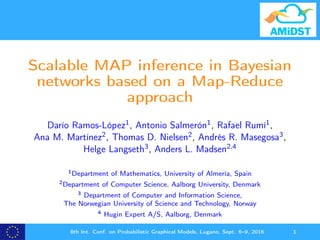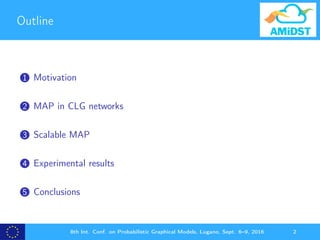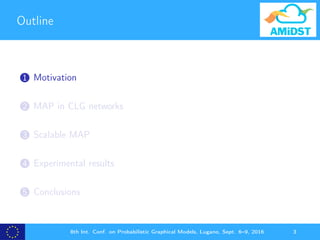Diese Präsentation wurde erfolgreich gemeldet.

# Scalable MAP inference in Bayesian networks based on a Map-Reduce approach (PGM2016)

Anzeige
Anzeige
Anzeige
Anzeige
Anzeige
Anzeige
Anzeige
Anzeige
Anzeige
Anzeige
Anzeige×

1 von 35 Anzeige

# Scalable MAP inference in Bayesian networks based on a Map-Reduce approach (PGM2016)

Maximum a posteriori (MAP) inference is a particularly complex type of probabilistic inference in Bayesian networks. It consists of finding the most probable configuration of a set of variables of interest given observations on a collection of other variables. In this paper we study scalable solutions to the MAP problem in hybrid Bayesian networks parameterized using conditional linear Gaussian distributions. We propose scalable solutions based on hill climbing and simulated anneal- ing, built on the Apache Flink framework for big data processing. We analyze the scalability of the solution through a series of experiments on large synthetic networks.

Full text paper: http://www.jmlr.org/proceedings/papers/v52/ramos-lopez16.pdf

Maximum a posteriori (MAP) inference is a particularly complex type of probabilistic inference in Bayesian networks. It consists of finding the most probable configuration of a set of variables of interest given observations on a collection of other variables. In this paper we study scalable solutions to the MAP problem in hybrid Bayesian networks parameterized using conditional linear Gaussian distributions. We propose scalable solutions based on hill climbing and simulated anneal- ing, built on the Apache Flink framework for big data processing. We analyze the scalability of the solution through a series of experiments on large synthetic networks.

Full text paper: http://www.jmlr.org/proceedings/papers/v52/ramos-lopez16.pdf

Anzeige
Anzeige

### Scalable MAP inference in Bayesian networks based on a Map-Reduce approach (PGM2016)

1. 1. Scalable MAP inference in Bayesian networks based on a Map-Reduce approach Darío Ramos-López1, Antonio Salmerón1, Rafael Rumí1, Ana M. Martínez2, Thomas D. Nielsen2, Andrés R. Masegosa3, Helge Langseth3, Anders L. Madsen2,4 1Department of Mathematics, University of Almería, Spain 2Department of Computer Science, Aalborg University, Denmark 3 Department of Computer and Information Science, The Norwegian University of Science and Technology, Norway 4 Hugin Expert A/S, Aalborg, Denmark 8th Int. Conf. on Probabilistic Graphical Models, Lugano, Sept. 6–9, 2016 1
2. 2. Outline 1 Motivation 2 MAP in CLG networks 3 Scalable MAP 4 Experimental results 5 Conclusions 8th Int. Conf. on Probabilistic Graphical Models, Lugano, Sept. 6–9, 2016 2
3. 3. Outline 1 Motivation 2 MAP in CLG networks 3 Scalable MAP 4 Experimental results 5 Conclusions 8th Int. Conf. on Probabilistic Graphical Models, Lugano, Sept. 6–9, 2016 3
4. 4. Motivation Aim: Provide scalable solutions to the MAP problem. Challenges: Data coming in streams at high speed, and a quick response is required. For each observation in the stream, the most likely conﬁguration of a set of variables of interest is sought. MAP inference is highly complex. Hybrid models come along with speciﬁc diﬃculties. 8th Int. Conf. on Probabilistic Graphical Models, Lugano, Sept. 6–9, 2016 4
5. 5. Context The AMiDST project: Analysis of MassIve Data STreams http://www.amidst.eu Large number of variables Queries to be answered in real time Hybrid Bayesian networks (involving discrete and continuous variables) Conditional linear Gaussian networks 8th Int. Conf. on Probabilistic Graphical Models, Lugano, Sept. 6–9, 2016 5
6. 6. Outline 1 Motivation 2 MAP in CLG networks 3 Scalable MAP 4 Experimental results 5 Conclusions 8th Int. Conf. on Probabilistic Graphical Models, Lugano, Sept. 6–9, 2016 6
7. 7. Conditional Linear Gaussian networks Y W TU S P(Y ) = (0.5, 0.5) P(S) = (0.1, 0.9) f (w|Y = 0) = N(w; −1, 1) f (w|Y = 1) = N(w; 2, 1) f (t|w, S = 0) = N(t; −w, 1) f (t|w, S = 1) = N(t; w, 1) f (u|w) = N(u; w, 1) 8th Int. Conf. on Probabilistic Graphical Models, Lugano, Sept. 6–9, 2016 7
8. 8. Querying a Bayesian network Belief update: Computing the posterior distribution of a variable: p(xi |xE ) = xD xC p(x, xE )dxC xDi xCi p(x, xE )dxCi Maximum a posteriori (MAP): For a set of target variables XI , seek x∗ I = arg max xI p(xI |XE = xE ) where p(xI |XE = xE ) is obtained by ﬁrst marginalizing out from p(x) the variables not in XI and not in XE Most probable explanation (MPE): A particular case of MAP where XI includes all the unobserved variables 8th Int. Conf. on Probabilistic Graphical Models, Lugano, Sept. 6–9, 2016 8
9. 9. Querying a Bayesian network Belief update: Computing the posterior distribution of a variable: p(xi |xE ) = xD xC p(x, xE )dxC xDi xCi p(x, xE )dxCi Maximum a posteriori (MAP): For a set of target variables XI , seek x∗ I = arg max xI p(xI |XE = xE ) where p(xI |XE = xE ) is obtained by ﬁrst marginalizing out from p(x) the variables not in XI and not in XE Most probable explanation (MPE): A particular case of MAP where XI includes all the unobserved variables 8th Int. Conf. on Probabilistic Graphical Models, Lugano, Sept. 6–9, 2016 8
10. 10. Outline 1 Motivation 2 MAP in CLG networks 3 Scalable MAP 4 Experimental results 5 Conclusions 8th Int. Conf. on Probabilistic Graphical Models, Lugano, Sept. 6–9, 2016 9
11. 11. MAP by Hill Climbing HC_MAP(B,xI ,xE ,r) while stopping criterion not satisﬁed do x∗ I ← GenerateConﬁguration(B, xI , xE , r) if p(x∗ I , xE ) ≥ p(xI , xE ) then xI ← x∗ I end end return xI 8th Int. Conf. on Probabilistic Graphical Models, Lugano, Sept. 6–9, 2016 10
12. 12. MAP by Hill Climbing HC_MAP(B,xI ,xE ,r) while stopping criterion not satisﬁed do x∗ I ← GenerateConﬁguration(B, xI , xE , r) if p(x∗ I , xE ) ≥ p(xI , xE ) then xI ← x∗ I end end return xI Max. number of non-improving iterations Target prob. threshold Max. number of iterations 8th Int. Conf. on Probabilistic Graphical Models, Lugano, Sept. 6–9, 2016 10
13. 13. MAP by Hill Climbing HC_MAP(B,xI ,xE ,r) while stopping criterion not satisﬁed do x∗ I ← GenerateConﬁguration(B, xI , xE , r) if p(x∗ I , xE ) ≥ p(xI , xE ) then xI ← x∗ I end end return xI Never move to a worse conﬁguration 8th Int. Conf. on Probabilistic Graphical Models, Lugano, Sept. 6–9, 2016 10
14. 14. MAP by Hill Climbing HC_MAP(B,xI ,xE ,r) while stopping criterion not satisﬁed do x∗ I ← GenerateConﬁguration(B, xI , xE , r) if p(x∗ I , xE ) ≥ p(xI , xE ) then xI ← x∗ I end end return xI Estimated using importance sampling: p(xI , xE ) = x∗∈ΩX∗ p(xI , xE , x∗ ) = x∗∈ΩX∗ p(xI , xE , x∗ ) f ∗(x∗) f ∗ (x∗ ) = Ef ∗ p(xI , xE , x∗ ) f ∗(x∗) ≈ 1 n n i=1 p xI , xE , x∗(i) f ∗ x∗(i) , 8th Int. Conf. on Probabilistic Graphical Models, Lugano, Sept. 6–9, 2016 10
15. 15. MAP by Hill Climbing HC_MAP(B,xI ,xE ,r) while stopping criterion not satisﬁed do x∗ I ← GenerateConﬁguration(B, xI , xE , r) if p(x∗ I , xE ) ≥ p(xI , xE ) then xI ← x∗ I end end return xI We’ll see later 8th Int. Conf. on Probabilistic Graphical Models, Lugano, Sept. 6–9, 2016 10
16. 16. MAP by Simulated Annealing SA_MAP(B,xI ,xE ,r) T ← 1 000; α ← 0.90; ε > 0 while T ≥ ε do x∗ I ← GenerateConﬁguration(B, xI , xE , r) Simulate a random number τ ∼ U(0, 1) if p(x∗ I , xE ) > p(xI , xE )/(T · ln(1/τ)) then xI ← x∗ I end T ← α · T end return xI 8th Int. Conf. on Probabilistic Graphical Models, Lugano, Sept. 6–9, 2016 11
17. 17. MAP by Simulated Annealing SA_MAP(B,xI ,xE ,r) T ← 1 000; α ← 0.90; ε > 0 while T ≥ ε do x∗ I ← GenerateConﬁguration(B, xI , xE , r) Simulate a random number τ ∼ U(0, 1) if p(x∗ I , xE ) > p(xI , xE )/(T · ln(1/τ)) then xI ← x∗ I end T ← α · T end return xI Default values of the temperature parameters T 1: almost completely random T 1: almost completely greedy 8th Int. Conf. on Probabilistic Graphical Models, Lugano, Sept. 6–9, 2016 11
18. 18. MAP by Simulated Annealing SA_MAP(B,xI ,xE ,r) T ← 1 000; α ← 0.90; ε > 0 while T ≥ ε do x∗ I ← GenerateConﬁguration(B, xI , xE , r) Simulate a random number τ ∼ U(0, 1) if p(x∗ I , xE ) > p(xI , xE )/(T · ln(1/τ)) then xI ← x∗ I end T ← α · T end return xI Accept x∗ I if its prob. increases or decreases < T · ln(1/τ) 8th Int. Conf. on Probabilistic Graphical Models, Lugano, Sept. 6–9, 2016 11
19. 19. MAP by Simulated Annealing SA_MAP(B,xI ,xE ,r) T ← 1 000; α ← 0.90; ε > 0 while T ≥ ε do x∗ I ← GenerateConﬁguration(B, xI , xE , r) Simulate a random number τ ∼ U(0, 1) if p(x∗ I , xE ) > p(xI , xE )/(T · ln(1/τ)) then xI ← x∗ I end T ← α · T end return xI Cool down the temperature 8th Int. Conf. on Probabilistic Graphical Models, Lugano, Sept. 6–9, 2016 11
20. 20. Generating a new conﬁguration of variables Only discrete variables The new values are chosen at random 8th Int. Conf. on Probabilistic Graphical Models, Lugano, Sept. 6–9, 2016 12
21. 21. Generating a new conﬁguration of variables Hybrid models We take advantage of the properties of the CLG distribution 8th Int. Conf. on Probabilistic Graphical Models, Lugano, Sept. 6–9, 2016 12
22. 22. Generating a new conﬁguration of variables Hybrid models We take advantage of the properties of the CLG distribution A variable whose parents are discrete or observed Y W TU S P(Y ) = (0.5, 0.5) P(S) = (0.1, 0.9) f (w|Y = 0) = N(w; −1, 1) f (w|Y = 1) = N(w; 2, 1) f (t|w, S = 0) = N(t; −w, 1) f (t|w, S = 1) = N(t; w, 1) f (u|w) = N(u; w, 1) 8th Int. Conf. on Probabilistic Graphical Models, Lugano, Sept. 6–9, 2016 12
23. 23. Generating a new conﬁguration of variables Hybrid models We take advantage of the properties of the CLG distribution A variable whose parents are discrete or observed Y W TU S Return its conditional mean: f (w|Y = 0) = N(w; −1, 1) f (w|Y = 1) = N(w; 2, 1) 8th Int. Conf. on Probabilistic Graphical Models, Lugano, Sept. 6–9, 2016 13
24. 24. Generating a new conﬁguration of variables Hybrid models We take advantage of the properties of the CLG distribution A variable with unobserved continuous parents Y W TU S Simulate a value using its conditional distribution: f (t|w, S = 0) = N(t; −w, 1) f (t|w, S = 1) = N(t; w, 1) 8th Int. Conf. on Probabilistic Graphical Models, Lugano, Sept. 6–9, 2016 13
25. 25. Scalable implementation 8th Int. Conf. on Probabilistic Graphical Models, Lugano, Sept. 6–9, 2016 14
26. 26. Outline 1 Motivation 2 MAP in CLG networks 3 Scalable MAP 4 Experimental results 5 Conclusions 8th Int. Conf. on Probabilistic Graphical Models, Lugano, Sept. 6–9, 2016 15
27. 27. Experimental analysis Purpose Analyze the scalability in terms of Speed Accuracy 8th Int. Conf. on Probabilistic Graphical Models, Lugano, Sept. 6–9, 2016 16
28. 28. Experimental analysis Purpose Analyze the scalability in terms of Speed Accuracy Experimental setup Synthetic networks with 200 variables (50% discrete) 70% of the variables observed at random 10% of the variables selected as target ⇒ 20% to be marginalized out 8th Int. Conf. on Probabilistic Graphical Models, Lugano, Sept. 6–9, 2016 16
29. 29. Experimental analysis Computing environment AMIDST Toolbox with Apache Flink Multi-core environment based on a dual-processor AMD Opteron 2.8 GHz server with 32 cores and 64 GB of RAM, running Ubuntu Linux 14.04.1 LTS Multi-node environment based on Amazon Web Services (AWS) 8th Int. Conf. on Probabilistic Graphical Models, Lugano, Sept. 6–9, 2016 17
30. 30. Scalability: run times 100 200 300 400 500 600 1 2 4 8 16 24 32 Number of cores Executiontime(s) method HC Global HC Local SA Global SA Local Scalability of MAP in a multi−core node 5 10 15 Speed-upfactor 5 10 15 20 1 2 4 8 16 24 32 Number of nodes (4 vCPUs per node)Speed−upfactor method HC Global HC Local SA Global SA Local Scalability of MAP in a multi−node cluster 25 50 75 100 125 1 2 4 8 16 24 32 Number of nodes (4 vCPUs per node)Executiontime(s) method HC Global HC Local SA Global SA Local Scalability of MAP in a multi−node cluster 5 10 15 20 Speed-upfactor 8th Int. Conf. on Probabilistic Graphical Models, Lugano, Sept. 6–9, 2016 18
31. 31. Scalability: accuracy (Simulated Annealing) −230 −220 −210 −200 −190 1 2 4 8 16 32 Number of cores logProbability cores 1 2 4 8 16 32 Simulated Annealing global −200 −195 −190 −185 1 2 4 8 16 32 Number of cores logProbability cores 1 2 4 8 16 32 Simulated Annealing local Estimated log-probabilities of the MAP conﬁgurations found by each algorithm 8th Int. Conf. on Probabilistic Graphical Models, Lugano, Sept. 6–9, 2016 19
32. 32. Scalability: accuracy (Hill Climbing) −240 −220 −200 1 2 4 8 16 32 Number of cores logProbability cores 1 2 4 8 16 32 Hill Climbing global −240 −220 −200 1 2 4 8 16 32 Number of cores logProbability cores 1 2 4 8 16 32 Hill Climbing local Estimated log-probabilities of the MAP conﬁgurations found by each algorithm 8th Int. Conf. on Probabilistic Graphical Models, Lugano, Sept. 6–9, 2016 20
33. 33. Outline 1 Motivation 2 MAP in CLG networks 3 Scalable MAP 4 Experimental results 5 Conclusions 8th Int. Conf. on Probabilistic Graphical Models, Lugano, Sept. 6–9, 2016 21
34. 34. Conclusions Scalable MAP for CLG models in terms of accuracy and run time Available in the AMIDST Toolbox Valid for multi-cores and cluster systems MapReduce-based design on top of Apache Flink 8th Int. Conf. on Probabilistic Graphical Models, Lugano, Sept. 6–9, 2016 22
35. 35. Thank you for your attention You can download our open source Java toolbox: http://www.amidsttoolbox.com Acknowledgments: This project has received funding from the European Union’s Seventh Framework Programme for research, technological development and demonstration under grant agreement no 619209 8th Int. Conf. on Probabilistic Graphical Models, Lugano, Sept. 6–9, 2016 23